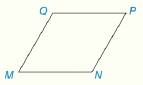Chapter 1.CR, Problem 14CR### Elementary Geometry for College St...

6th Edition
Daniel C. Alexander + 1 other
ISBN: 9781285195698

#### Solutions

Chapter
Section### Elementary Geometry for College St...

6th Edition
Daniel C. Alexander + 1 other
ISBN: 9781285195698
Textbook Problem
17 views

# Figure M N P Q is a rhombus. Draw diagonals M P ¯ and Q N ¯ of the rhombus. How do M P ¯ and Q N ¯ appear to be related?To determine

To plot:

The diagonals MP¯ and QN¯ of a given rhombus and find the relation between MP¯ and QN¯.

Explanation

Given:

The Figure (1) of the rhombus MNPQ is given as,

Figure (1)

Approach:

In a rhombus the diagonals are perpendicular to each other.

Draw the diagonal QN by joining a line from Q to N.

Draw the diagonal MP by joining a line from M to P

### Still sussing out bartleby?

Check out a sample textbook solution.

See a sample solution

#### The Solution to Your Study Problems

Bartleby provides explanations to thousands of textbook problems written by our experts, many with advanced degrees!

Get Started

#### Convert the expressions in Exercises 8596 radical form. y7/4

Finite Mathematics and Applied Calculus (MindTap Course List)

#### In Problems 33 – 38, solve each inequality. 34.

Mathematical Applications for the Management, Life, and Social Sciences

#### True or False: The domain is all numbers x for which f(x) is defined.

Study Guide for Stewart's Single Variable Calculus: Early Transcendentals, 8th

#### Find if , y = sin 2t.

Study Guide for Stewart's Multivariable Calculus, 8th